5. Addition of sound intensitiesObjectives
1. Understanding the principles of adding two sounds together
2. Understand how to convert dB SPL or dB IL values to intensities prior to adding
3. Understand how to how to combine octave or 1/3rd octave measures
4. Understand what is meant by spectrum level.
5. Understand how to combine sound exposures over time.

5.1 Conversion from dB intensity level (dB IL) to sound intensity

Because the decibel scale is a logarithmic scale, if we have two different sound sources combining together we can't simply add the sound pressure levels - what we have to do is to add the intensities. That means we have to convert our decibel values into intensities (with units of W/m2).

Remember that if we have an sound intensity (I) an wish to express it in dB intensity level we need to use: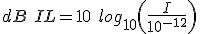What we need is an expression for intensity (I). So let us now rearrange the equations to get an I on its own.

To do this we first divide by 10 on both sides: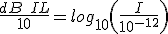Then take the inverse log (antilog) of both sides: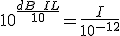then we multiply both sides by the reference intensity to get  I on its own: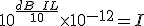(5.1)Reflection
Example 1. Using equation  5.1, if a  sound is 80 dB IL, what is the sound intensity?
5.2 Adding two equal sound intensities

So, for example suppose we have two independent sound sources producing white-noise and the sound pressure level of each one measured on it's own is 80 dB SPL - our question is, what is the resulting sound pressure level when they are both turned on together?

If we assume that the value in dB SPL is the same as it would be if we measured it in dB IL (i.e. assumptions of a plane wave) then the first thing we need to do is convert our dB SPLs into intensities as in 5.1. (see Example 1). If we refer to the two sound intensities as I1 and I2 which are both equal, then as we have already seen: I1 = I2 = 10-4 W/m2

If we now add I1 and I2  to give Itotal we have:

Itotal = I1 + I2 = 10-4 + 10-4=2 x 10-4 W/m2

So we now have the sound intensity of our combined signal and we can now convert this back to a dB value: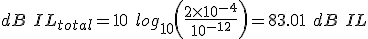Now since we are talking about plane waves, our total sould pressure level = 83.01 dB SPL.

This example gives us a simple rule:

If we add two unrelated sounds of the same intensity together, it is equivalent to a 3 dB increase in the total sound pressure level.Reflection
Example 2. Given the above example, what do you think the total sound pressure level would be if 4 unrelated white-noise sound sources each of 80 dB SPL were combined?
5.3 Adding two unequal sound intensities

Suppose we have two unrelated sound sources of unequal SPLs (e.g. 80 and 74 dB SPL. Using the above procedure we can show that the two intensities are 10-4 and 2.5x10-5 W/m2 respectively. If we add these together we get 1.25x10-4 W/m2 which if we convert back to decibels gives us approximately 81 dB SPL. The lower level sound only makes a relatively small additional contribution to the total sound pressure level.

We can extend this process further if we want to add more than two unrelated sound levels and this would lead to the general equation for a set of sound levels L1, L2, ... Ln: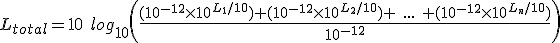(5.2)

But this can be simplified since the reference intensities cancel out to give: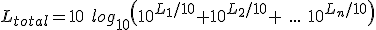(5.3)

5.4 Nomogram for adding sound levels

Various people have come up with simple ways of short-cutting these procedures - figure 5.1 shows one way of doing this for two sound levels.

Figure 5.1: Nomogram for the addition of two decibel levels. (1) Calculate the difference between the two sound levels and select this value on the upper scale (2) read off the value on the lower scale and add this to the higher of the two sound levels.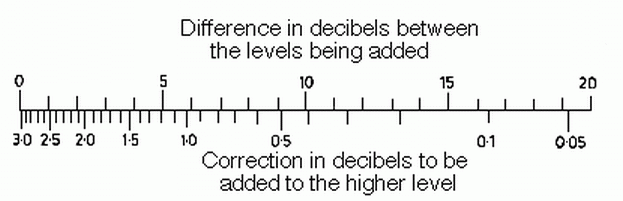Figure 5.2: Nomogram for addition of decibels

So for our previous example, we take the difference between the two sound levels (80 - 74 = 6 dB) and read the lower scale to find the correction (approx. 1 dB) this is then added to the higher sound level (i.e. 80+1 = 81 dB SPL).

At the left hand side of the nomogram, if the two sound levels are equal (difference = zero) then we should add 3 dB (i.e. doubling the sound intensity). At the right hand of the scales, if the two sound levels differ by as much as 20dB then the lower sound level makes very little difference to the total sound level.

If we have more than two sound levels to add we can simply break them down into a series of pairs. For example if we have 3 measurements of 80.8, 83 and 84 dB SPL. We can add the 80.8 and 83 first to give approx. 85 dB SPL and then add this to the 84 dB SPL which would give us a total of approximately 87.5 dB SPL. If we were to calculate this using equation (5.3) we would get 87.6 dB SPL - try this for yourself.

5.4 Why Unrelated Sounds?

In the above descriptions it is emphasized that we are concerned with adding unrelated sounds - the reason for this is that if two sound waveforms are related (e.g. two sinusoids of the same frequency) then we have to take into account the actual phases as well as the amplitudes.

For example if we have two speakers producing a sinusoid of the same amplitude and frequency the actual amplitude of the combines signal will depend on the relative phases. If they are 180 degrees out of phase they will cancel out (destructive interference) and the resulting sound level will be zero. If they are in phase with each other then their amplitudes (sound pressures) will add (constructive interference). In this case the sound pressure will double so the sound level will increase by 6 dB (i.e. 20 log10(2)).

So if we are adding two sound from the same source or sounds with waveforms that are similar then we have to think carefully before adding them together.